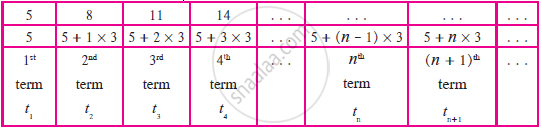# General Term of an Arithmetic Progression

## Notes

In the sequence 5, 8, 11, 14, . . . The difference between two consecutive terms is 3.
Hence, this sequence is an A.P.
Here, the first term is 5. If 3 is added to 5, we get the second term 8. Similarly, to find the 100th term, what should be done?

First term       Second term         Third term . . .
Number 5,     5 + 3 = 8                8 + 3 = 11 . . .
In this way, reaching up to the 100th term will be time-consuming. Let’s see if we can find any formula for it.Generally in the A.P. t1, t2, t3, . . . If the first term is a and the common difference is d,
t1= a
t2= t1+ d = a + d = a + (2 - 1) d
t3= t2+ d = a + d + d = a + 2d = a + (3 - 1)d
t4= t3+ d = a + 2d + d = a + 3d = a +(4 - 1)d
We get tn= a +(n - 1) d.

Using the above formula, we can find the 100th term of the A.P. 5, 8, 11, 14, . . .
Here a = 5 d = 3
tn = a +(n - 1)d
t100= 5 +(100 - 1) × 3
= 5 + 99 × 3
= 5 + 297
t100 = 302
The 100th term of this A.P. is 302.

If you would like to contribute notes or other learning material, please submit them using the button below.